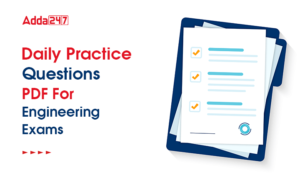Engineering Jobs   »   Quiz: Mechanical Engineering 06 May 2020

# Quiz: Mechanical Engineering 06 May 2020

Quiz: Mechanical Engineering
Exam: UPSSSC JE
Topic: Miscellaneous

Each question carries 1 mark
Negative marking: 1/4 mark
Time: 10 Minutes

Q1. A continuous beam is one which has
(a) Single support
(b) Two supports
(c) Both a and b
(d) More than two supports

Q2. The strength of beam, mainly depends upon
(a) Bending moment
(b) Beam weight
(c) Section modulus
(d) C.G. of the section

Q3. At the principle plane in the Mohr’s circle
(a) The shear stress is maximum
(b) The shear stress is zero
(c) The normal stress is zero
(d) Tensile stress is zero and shear stress is maximum

Q4. If the stiffness of the spring is increased then Hartnell’s governor will become
(a) Hunting
(b) Isochronous
(c) More sensitive
(d) Less sensitive

Q5. In Carnot cycle heat is rejected at
(a) Constant pressure
(b) Constant temperature
(c) Constant volume
(d) Constant Entropy

Q6. The diameter of the rivets in mm for a plate of thickness ‘t’ (mm) is taken as
(a) t
(b) 2t
(c) √t
(d) 6√t

(a) Cantilever beam
(b) Over-hanging beam
(c) Simply supported beam
(d) All of the above

Q8. A piezometric tube is used for measuring
(a) Low pressure
(b) High pressure
(c) Moderate pressure
(d) Vacuum pressure

Q9. Which of the following is boiler accessories?
(a) Safety valve
(b) Pressure gauge
(c) Water level indicator
(d) Superheater

Q10. Through of cam is the maximum distance of follower from-
(a) Base circle
(b) Pitch circle
(c) Root circle
(d) Prime circle

Solutions

S1. Ans.(d)
Sol. A continuous beam is one which is supported on more than two supports. A Cantilever beam is supported at one end. A simply supported beam is supported on two ends.

S2. Ans.(c)
Sol. The strength of beam mainly depends upon section modulus of the beam.
Section modulus is the ratio of moment of inertia of the beam and the maximum vertical distance from neutral axis at cross section or it may be defined as the ratio of total moment resisted by the section to the stress in the extreme fiber which is equal to yield stress.
M/I=σ/Y
Section modulus=M/σ=I/Y

S3. Ans.(b)
Sol. Principal planes are those planes in the Mohr’s circle, on which shear stress is zero or we can say normal stress is maximum on principal planes.

S4. Ans.(d)
Sol. As the stiffness of the spring is increased, then the sleeve movement is difficult which decreases the sensitiveness of the Hartnell governor for small change of speed.

S5. Ans.(b)
Sol. In Carnot cycle, there are four processes mainly.
2. Isentropic expansion
3. Constant temperature heat rejection
4. Isentropic compression

S6. Ans.(d)
Sol. According to Unwin’s formula
Diameter of the rivet (d)=6√t

S7. Ans.(b)
Sol. Point of contra-flexure is that point where bending moment diagram changes its sign or where shear stress is maximum. In normal loading conditions, we get point of contra-flexure in overhanging beams only.

S8. Ans.(a)
Sol. Due to restriction of length of the tube, piezometric tube is used for low pressure measurement only.

S9. Ans.(d)
Sol. Boiler accessories are that equipment that are connected with the boiler for increasing the performance and efficiency of the boiler. Such as-economizer, feed water pump, superheater, air pre-heater, steam trap etc.

S10. Ans.(a)
Sol. Through of cam is the maximum distance of the follower from the base circle.

Sharing is caring!

•GATE Result 2023 Out, Download Result, C...
•GATE 2023 Admit Card Out, Download Link ...
•UKPSC Draftsman Syllabus 2023 and Exam P...
•UP Polytechnic Exam Date 2023 Out, Downl...
•JSSC JE Syllabus 2023, Check Latest Exam...
•Daily Practice Questions PDF For Enginee...
•ISRO Recruitment 2023, Latest Notificati...
•UPSC ESE Previous Year Papers, Download ...